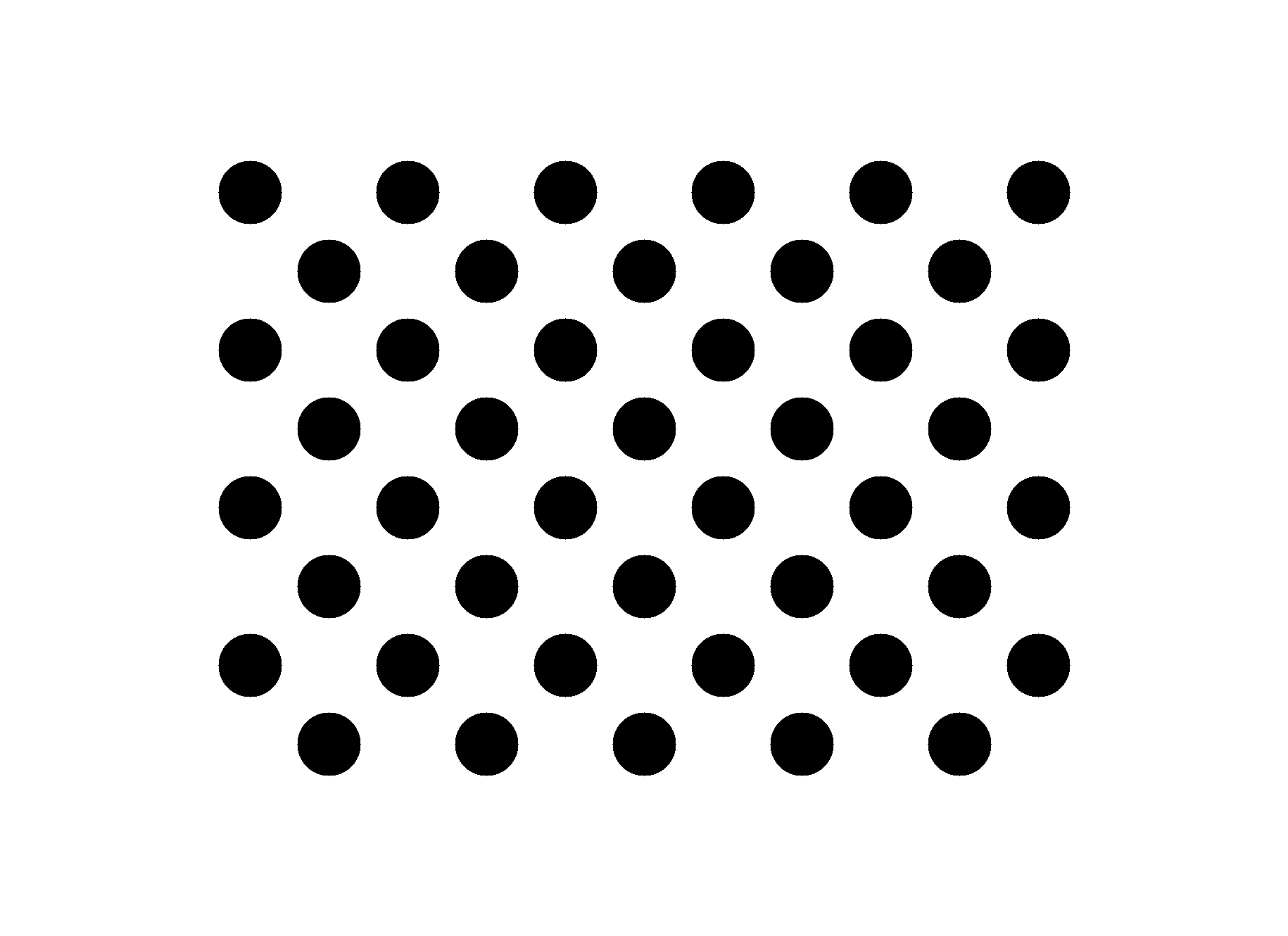# Preparation

A widely used asymmetric circle grid pattern can be found in doc of OpenCV 2.4. Same as previous blogs, the camera needs to be calibrated beforehand. For this asymmetric circle grid example, a sequence of images (instead of a video stream) is tested.

# Coding

The code can be found at OpenCV Examples.

## First of all

We need to ensure cv2.so is under our system path. cv2.so is specifically for OpenCV Python.

Then, we import some packages to be used (NO ArUco).

## Secondly

We now load all camera calibration parameters, including: cameraMatrix, distCoeffs, etc. For example, your code might look like the following:

Since we are testing a calibrated fisheye camera, two extra parameters are to be loaded from the calibration file.

Afterwards, two mapping matrices are pre-calculated by calling function cv2.fisheye.initUndistortRectifyMap() as (supposing the images to be processed are of 1080P):

## Thirdly

The circle pattern is to be loaded.Here in our case, this asymmetric circle grid pattern is manually loaded as follows:

In our case, the distance between two neighbour circle centres (in the same column) is measured as 72 centimetres. Meanwhile, the axis at the origin is loaded as well, with respective length 300, 200, 100 centimetres.

## Fourthly

Since we are going to use OpenCV’s SimpleBlobDetector for the blob detection, the SimpleBlobDetector’s parameters are to be created beforehand. The parameter values can be adjusted according to your own testing environments. The iteration criteria for the simple blob detection is also created at the same time.

## Finally

Estimate camera postures. Here, we are testing a sequence of images, rather than video streams. We first list all file names in sequence.

Then, we calculate the camera posture frame by frame:

The drawn axis is just the world coordinators and orientations estimated from the images taken by the testing camera.